# W3cubDocs

/TensorFlow Guide

# Convolutional Neural Networks

NOTE: This tutorial is intended for advanced users of TensorFlow and assumes expertise and experience in machine learning.

## Overview

CIFAR-10 classification is a common benchmark problem in machine learning. The problem is to classify RGB 32x32 pixel images across 10 categories:

```airplane, automobile, bird, cat, deer, dog, frog, horse, ship, and truck.
```

For more details refer to the CIFAR-10 page and a Tech Report by Alex Krizhevsky.

### Goals

The goal of this tutorial is to build a relatively small convolutional neural network (CNN) for recognizing images. In the process, this tutorial:

1. Highlights a canonical organization for network architecture, training and evaluation.
2. Provides a template for constructing larger and more sophisticated models.

The reason CIFAR-10 was selected was that it is complex enough to exercise much of TensorFlow's ability to scale to large models. At the same time, the model is small enough to train fast, which is ideal for trying out new ideas and experimenting with new techniques.

### Highlights of the Tutorial

The CIFAR-10 tutorial demonstrates several important constructs for designing larger and more sophisticated models in TensorFlow:

We also provide a multi-GPU version of the model which demonstrates:

• Configuring a model to train across multiple GPU cards in parallel.
• Sharing and updating variables among multiple GPUs.

We hope that this tutorial provides a launch point for building larger CNNs for vision tasks on TensorFlow.

### Model Architecture

The model in this CIFAR-10 tutorial is a multi-layer architecture consisting of alternating convolutions and nonlinearities. These layers are followed by fully connected layers leading into a softmax classifier. The model follows the architecture described by Alex Krizhevsky, with a few differences in the top few layers.

This model achieves a peak performance of about 86% accuracy within a few hours of training time on a GPU. Please see below and the code for details. It consists of 1,068,298 learnable parameters and requires about 19.5M multiply-add operations to compute inference on a single image.

## Code Organization

The code for this tutorial resides in `models/tutorials/image/cifar10/`.

File Purpose
`cifar10_input.py` Reads the native CIFAR-10 binary file format.
`cifar10.py` Builds the CIFAR-10 model.
`cifar10_train.py` Trains a CIFAR-10 model on a CPU or GPU.
`cifar10_multi_gpu_train.py` Trains a CIFAR-10 model on multiple GPUs.
`cifar10_eval.py` Evaluates the predictive performance of a CIFAR-10 model.

## CIFAR-10 Model

The CIFAR-10 network is largely contained in `cifar10.py`. The complete training graph contains roughly 765 operations. We find that we can make the code most reusable by constructing the graph with the following modules:

1. Model inputs: `inputs()` and `distorted_inputs()` add operations that read and preprocess CIFAR images for evaluation and training, respectively.
2. Model prediction: `inference()` adds operations that perform inference, i.e. classification, on supplied images.
3. Model training: `loss()` and `train()` add operations that compute the loss, gradients, variable updates and visualization summaries.

### Model Inputs

The input part of the model is built by the functions `inputs()` and `distorted_inputs()` which read images from the CIFAR-10 binary data files. These files contain fixed byte length records, so we use `tf.FixedLengthRecordReader`. See Reading Data to learn more about how the `Reader` class works.

The images are processed as follows:

• They are cropped to 24 x 24 pixels, centrally for evaluation or randomly for training.
• They are approximately whitened to make the model insensitive to dynamic range.

For training, we additionally apply a series of random distortions to artificially increase the data set size:

Please see the Images page for the list of available distortions. We also attach an `tf.summary.image` to the images so that we may visualize them in TensorBoard. This is a good practice to verify that inputs are built correctly.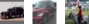Reading images from disk and distorting them can use a non-trivial amount of processing time. To prevent these operations from slowing down training, we run them inside 16 separate threads which continuously fill a TensorFlow queue.

### Model Prediction

The prediction part of the model is constructed by the `inference()` function which adds operations to compute the logits of the predictions. That part of the model is organized as follows:

Layer Name Description
`conv1` convolution and rectified linear activation.
`pool1` max pooling.
`norm1` local response normalization.
`conv2` convolution and rectified linear activation.
`norm2` local response normalization.
`pool2` max pooling.
`local3` fully connected layer with rectified linear activation.
`local4` fully connected layer with rectified linear activation.
`softmax_linear` linear transformation to produce logits.

Here is a graph generated from TensorBoard describing the inference operation: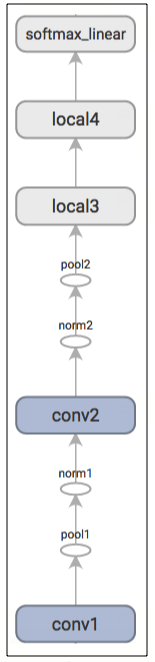EXERCISE: The output of `inference` are un-normalized logits. Try editing the network architecture to return normalized predictions using `tf.nn.softmax`.

The `inputs()` and `inference()` functions provide all the components necessary to perform an evaluation of a model. We now shift our focus towards building operations for training a model.

EXERCISE: The model architecture in `inference()` differs slightly from the CIFAR-10 model specified in cuda-convnet. In particular, the top layers of Alex's original model are locally connected and not fully connected. Try editing the architecture to exactly reproduce the locally connected architecture in the top layer.

### Model Training

The usual method for training a network to perform N-way classification is multinomial logistic regression, aka. softmax regression. Softmax regression applies a softmax nonlinearity to the output of the network and calculates the cross-entropy between the normalized predictions and the label index. For regularization, we also apply the usual weight decay losses to all learned variables. The objective function for the model is the sum of the cross entropy loss and all these weight decay terms, as returned by the `loss()` function.

We visualize it in TensorBoard with a `tf.summary.scalar`: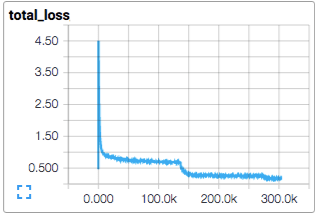We train the model using standard gradient descent algorithm (see Training for other methods) with a learning rate that exponentially decays over time.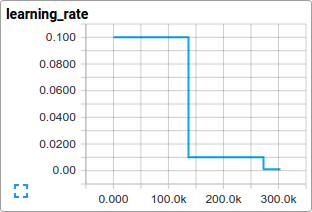The `train()` function adds the operations needed to minimize the objective by calculating the gradient and updating the learned variables (see `tf.train.GradientDescentOptimizer` for details). It returns an operation that executes all the calculations needed to train and update the model for one batch of images.

## Launching and Training the Model

We have built the model, let's now launch it and run the training operation with the script `cifar10_train.py`.

```python cifar10_train.py
```

NOTE: The first time you run any target in the CIFAR-10 tutorial, the CIFAR-10 dataset is automatically downloaded. The data set is ~160MB so you may want to grab a quick cup of coffee for your first run.

You should see the output:

```Filling queue with 20000 CIFAR images before starting to train. This will take a few minutes.
2015-11-04 11:45:45.927302: step 0, loss = 4.68 (2.0 examples/sec; 64.221 sec/batch)
2015-11-04 11:45:49.133065: step 10, loss = 4.66 (533.8 examples/sec; 0.240 sec/batch)
2015-11-04 11:45:51.397710: step 20, loss = 4.64 (597.4 examples/sec; 0.214 sec/batch)
2015-11-04 11:45:54.446850: step 30, loss = 4.62 (391.0 examples/sec; 0.327 sec/batch)
2015-11-04 11:45:57.152676: step 40, loss = 4.61 (430.2 examples/sec; 0.298 sec/batch)
2015-11-04 11:46:00.437717: step 50, loss = 4.59 (406.4 examples/sec; 0.315 sec/batch)
...
```

The script reports the total loss every 10 steps as well as the speed at which the last batch of data was processed. A few comments:

• The first batch of data can be inordinately slow (e.g. several minutes) as the preprocessing threads fill up the shuffling queue with 20,000 processed CIFAR images.

• The reported loss is the average loss of the most recent batch. Remember that this loss is the sum of the cross entropy and all weight decay terms.

• Keep an eye on the processing speed of a batch. The numbers shown above were obtained on a Tesla K40c. If you are running on a CPU, expect slower performance.

EXERCISE: When experimenting, it is sometimes annoying that the first training step can take so long. Try decreasing the number of images that initially fill up the queue. Search for `min_fraction_of_examples_in_queue` in `cifar10_input.py`.

`cifar10_train.py` periodically saves all model parameters in checkpoint files but it does not evaluate the model. The checkpoint file will be used by `cifar10_eval.py` to measure the predictive performance (see Evaluating a Model below).

If you followed the previous steps, then you have now started training a CIFAR-10 model. Congratulations!

The terminal text returned from `cifar10_train.py` provides minimal insight into how the model is training. We want more insight into the model during training:

• Is the loss really decreasing or is that just noise?
• Is the model being provided appropriate images?
• Are the gradients, activations and weights reasonable?
• What is the learning rate currently at?

TensorBoard provides this functionality, displaying data exported periodically from `cifar10_train.py` via a `tf.summary.FileWriter`.

For instance, we can watch how the distribution of activations and degree of sparsity in `local3` features evolve during training: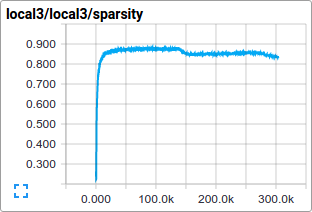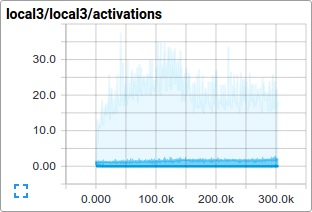Individual loss functions, as well as the total loss, are particularly interesting to track over time. However, the loss exhibits a considerable amount of noise due to the small batch size employed by training. In practice we find it extremely useful to visualize their moving averages in addition to their raw values. See how the scripts use `tf.train.ExponentialMovingAverage` for this purpose.

## Evaluating a Model

Let us now evaluate how well the trained model performs on a hold-out data set. The model is evaluated by the script `cifar10_eval.py`. It constructs the model with the `inference()` function and uses all 10,000 images in the evaluation set of CIFAR-10. It calculates the precision at 1: how often the top prediction matches the true label of the image.

To monitor how the model improves during training, the evaluation script runs periodically on the latest checkpoint files created by the `cifar10_train.py`.

```python cifar10_eval.py
```

Be careful not to run the evaluation and training binary on the same GPU or else you might run out of memory. Consider running the evaluation on a separate GPU if available or suspending the training binary while running the evaluation on the same GPU.

You should see the output:

```2015-11-06 08:30:44.391206: precision @ 1 = 0.860
...
```

The script merely returns the precision @ 1 periodically -- in this case it returned 86% accuracy. `cifar10_eval.py` also exports summaries that may be visualized in TensorBoard. These summaries provide additional insight into the model during evaluation.

The training script calculates the moving average version of all learned variables. The evaluation script substitutes all learned model parameters with the moving average version. This substitution boosts model performance at evaluation time.

EXERCISE: Employing averaged parameters may boost predictive performance by about 3% as measured by precision @ 1. Edit `cifar10_eval.py` to not employ the averaged parameters for the model and verify that the predictive performance drops.

## Training a Model Using Multiple GPU Cards

Modern workstations may contain multiple GPUs for scientific computation. TensorFlow can leverage this environment to run the training operation concurrently across multiple cards.

Training a model in a parallel, distributed fashion requires coordinating training processes. For what follows we term model replica to be one copy of a model training on a subset of data.

Naively employing asynchronous updates of model parameters leads to sub-optimal training performance because an individual model replica might be trained on a stale copy of the model parameters. Conversely, employing fully synchronous updates will be as slow as the slowest model replica.

In a workstation with multiple GPU cards, each GPU will have similar speed and contain enough memory to run an entire CIFAR-10 model. Thus, we opt to design our training system in the following manner:

• Place an individual model replica on each GPU.
• Update model parameters synchronously by waiting for all GPUs to finish processing a batch of data.

Here is a diagram of this model: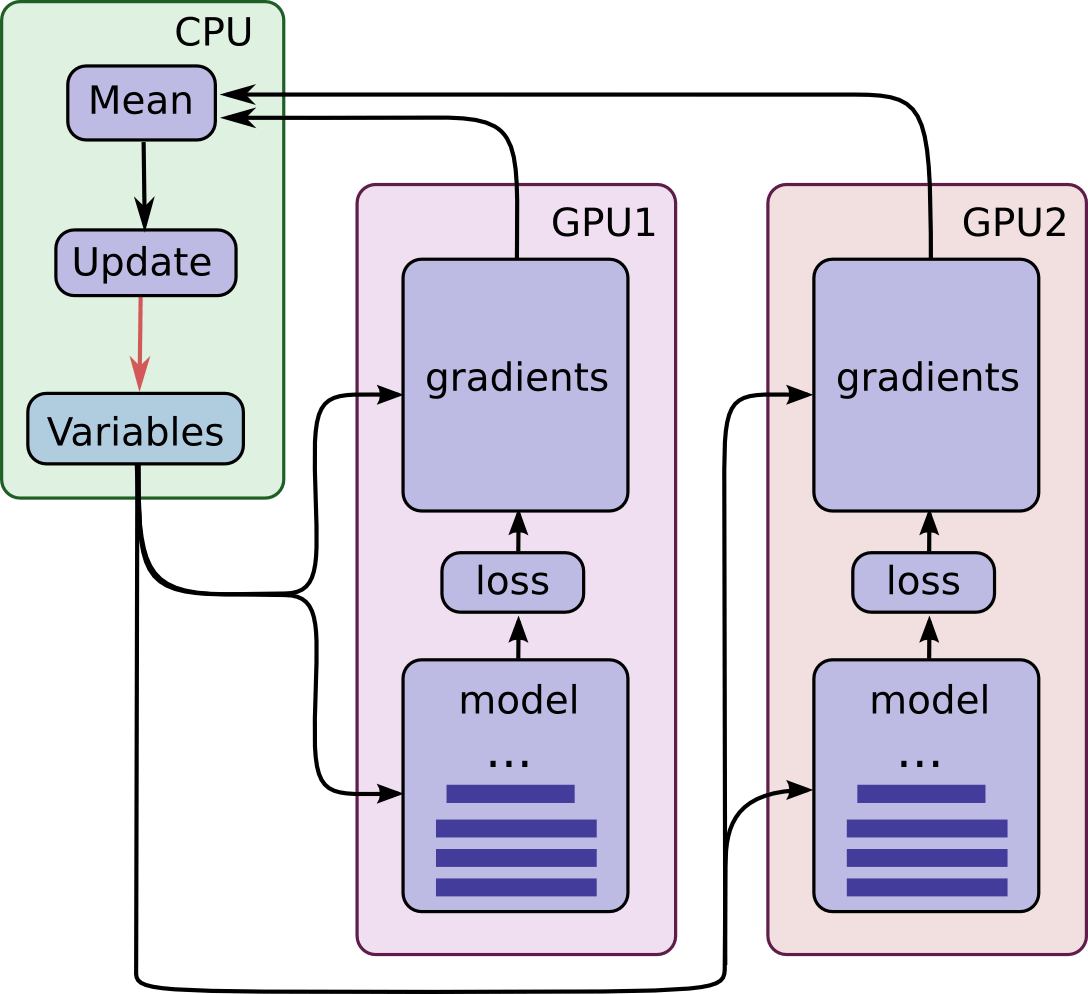Note that each GPU computes inference as well as the gradients for a unique batch of data. This setup effectively permits dividing up a larger batch of data across the GPUs.

This setup requires that all GPUs share the model parameters. A well-known fact is that transferring data to and from GPUs is quite slow. For this reason, we decide to store and update all model parameters on the CPU (see green box). A fresh set of model parameters is transferred to the GPU when a new batch of data is processed by all GPUs.

The GPUs are synchronized in operation. All gradients are accumulated from the GPUs and averaged (see green box). The model parameters are updated with the gradients averaged across all model replicas.

### Placing Variables and Operations on Devices

Placing operations and variables on devices requires some special abstractions.

The first abstraction we require is a function for computing inference and gradients for a single model replica. In the code we term this abstraction a "tower". We must set two attributes for each tower:

• A unique name for all operations within a tower. `tf.name_scope` provides this unique name by prepending a scope. For instance, all operations in the first tower are prepended with `tower_0`, e.g. `tower_0/conv1/Conv2D`.

• A preferred hardware device to run the operation within a tower. `tf.device` specifies this. For instance, all operations in the first tower reside within `device('/device:GPU:0')` scope indicating that they should be run on the first GPU.

All variables are pinned to the CPU and accessed via `tf.get_variable` in order to share them in a multi-GPU version. See how-to on Sharing Variables.

### Launching and Training the Model on Multiple GPU cards

If you have several GPU cards installed on your machine you can use them to train the model faster with the `cifar10_multi_gpu_train.py` script. This version of the training script parallelizes the model across multiple GPU cards.

```python cifar10_multi_gpu_train.py --num_gpus=2
```

Note that the number of GPU cards used defaults to 1. Additionally, if only 1 GPU is available on your machine, all computations will be placed on it, even if you ask for more.

EXERCISE: The default settings for `cifar10_train.py` is to run on a batch size of 128. Try running `cifar10_multi_gpu_train.py` on 2 GPUs with a batch size of 64 and compare the training speed.

## Next Steps

Congratulations! You have completed the CIFAR-10 tutorial.

If you are now interested in developing and training your own image classification system, we recommend forking this tutorial and replacing components to address your image classification problem.

EXERCISE: Download the Street View House Numbers (SVHN) data set. Fork the CIFAR-10 tutorial and swap in the SVHN as the input data. Try adapting the network architecture to improve predictive performance.

© 2018 The TensorFlow Authors. All rights reserved.
Licensed under the Creative Commons Attribution License 3.0.
Code samples licensed under the Apache 2.0 License.
https://www.tensorflow.org/tutorials/deep_cnn